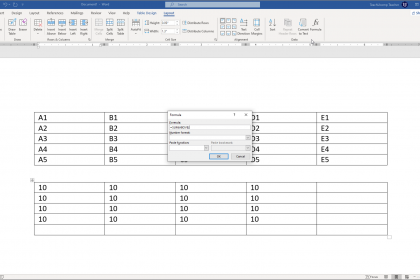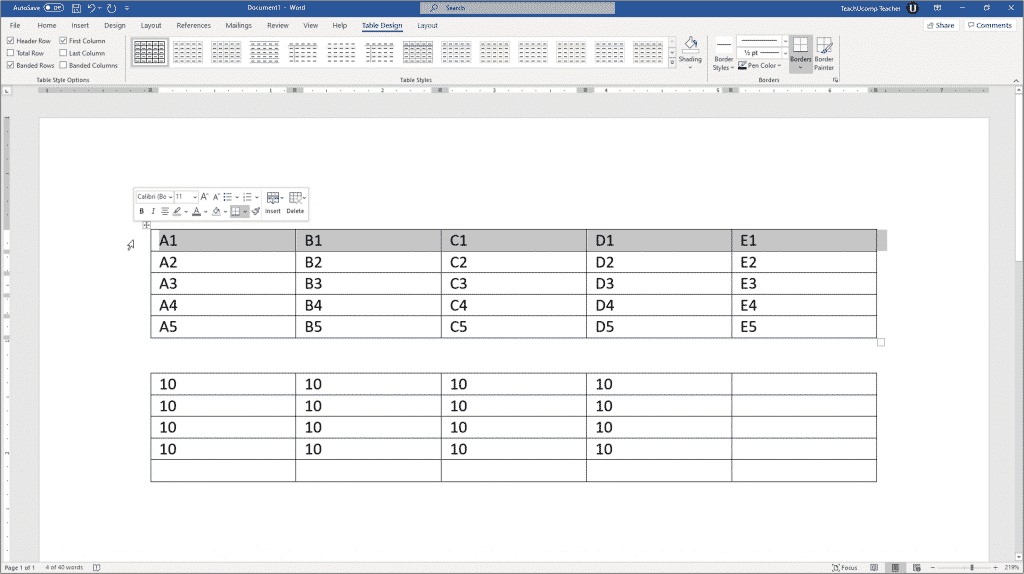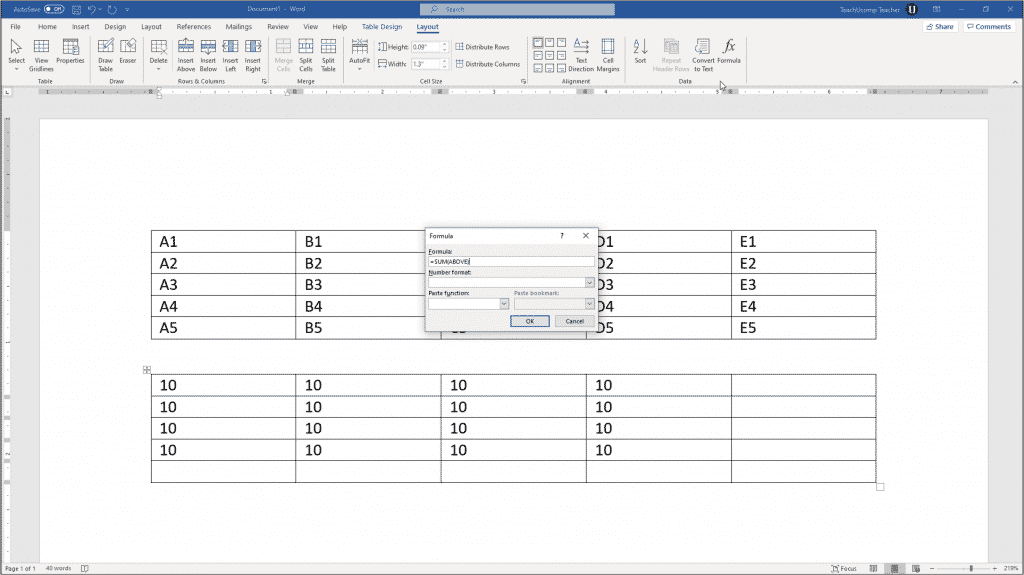# Insert Table Formulas in Word – Instructions

/ / Latest, Microsoft, Office 2013, Office 2016, Office 2019, Office 365, Word 2013, Word 2016, Word 2019, Word for Office 365## Insert Table Formulas in Word: Overview

You can insert table formulas in Word tables to perform simple mathematical functions on data. To insert table formulas in Word that add, subtract, multiply, and divide numbers in the table cells, you insert formulas into cells where you want to show the answers to the mathematical operations performed by the formulas.

### The Parts of Table Formulas in Word

When you insert table formulas in Word, you insert a field that performs calculations on values in other table cells. Formulas always start with an equal sign (=). They often refer to the cell addresses from which they gather the data for their calculations. These cell addresses can be linked together with standard mathematical operators. These include the plus sign (+), minus sign (-), multiplication sign (*), and division sign (/), among others. You can also perform functions, like SUM, on a cell range in a table. So, a formula might be expressed “=SUM(Above),” which adds the values of the cells above the cell into which you inserted this formula.

A cell address is a way of referring to a cell. A cell address is the relative location of a cell in a table. Imagine there are letters at the top of each column, starting with “A” at the far left and then continuing to increase one letter at a time to the right. In addition, imagine each row has a number assigned to it. The topmost row is row “1.” The row numbering then continues downward, increasing by one for each row. The cell address is the column letter, followed by the row number. For example, the top left cell is always cell A1. B1 is always to the right of A1. Here is a table with the cell addresses entered into the corresponding cells to help you see the cell address naming convention.###### Insert Table Formulas in Word – Instructions: A picture of the cell addresses within a sample table, shown at the top of the Word document.

As stated previously, when you insert table formulas in Word, you are creating a formula field. A cell formula begins with an equal sign (=). It is often followed by the cell addresses of the cells upon which to perform the mathematical operations, joined together by standard mathematical operators. For example, to add the cells above cell A5 and show the formula result in cell A5, click into cell A5. Then insert a formula field that looks like either: =A1+A2+A3+A4 or =SUM(Above).

Instead of showing the formula itself in the cell, the cell shows the answer to the formula. Why? Because when you insert table formulas in Word in a cell, Word knows it should show the answer to the formula, not the formula itself. Formulas display their results by default, not their actual contents.

### How to Insert Table Formulas in Word

To insert table formulas in Word into a table cell, click into the table cell where you want to show the answer. This is often the cell at the end of a continuous column or row of numbers. Next, click the table’s “Layout” contextual tab in the Ribbon. In older versions of Word, this appears as the “Layout” tab of the “Table Tools” contextual tab in the Ribbon. For all versions of Word, then click the “Formula” button in the “Data” button group to open the “Formula” dialog box. This dialog box lets you type the formulas used to insert table formulas in Word.

When the “Formula” dialog box first opens, Word tries to guess the formula you want. For example, if you insert table formulas in Word in a cell at the end of a column of continuous numbers, Word assumes you want to add the cell values in the column above the cell. Therefore, Word enters the formula =SUM(Above) as the default formula in the “Formula” dialog box.###### Insert Table Formulas in Word – Instructions: A picture of the default table formula that Word suggests in the “Formula” dialog box.

If Word suggests the correct formula, then click “OK” at the bottom of the “Formula” dialog box to accept it and insert the cell formula. If incorrect, then click into the “Formula:” field and enter the correct formula.

After entering the formula into the “Formula:” field, you can then use the “Number format:” drop-down to select a numeric pattern. This helps show the result in a specific numeric format.

In Word, you can use the terms “LEFT,” “RIGHT,” “ABOVE,” and “BELOW” to refer to adjacent cells in the row or column to the left of, to the right of, above, or below the cell within which you insert table formulas in Word. This is a convenient way of selecting the cell range for the function. You can also enter a cell range by typing the cell address of the upper-left cell in the cell range, followed by a colon symbol (:), then followed by the cell address of the lower-right cell in the range. For example, you could also type =SUM(A1:A4) into the “Formula:” field to add the contents of cells A1 through A4.

The word SUM is a formula function. If want to perform one mathematical operation on a range of cells, you can use functions like SUM, AVERAGE, MAX, and MIN when you insert table formulas in Word, instead of individually writing the cell addresses and mathematical operators. Word provides many standard functions in the “Paste function:” drop-down. Selecting any function from the list of functions in the drop-down menu adds it to the formula in the “Formula:” field.

After creating the Word formula, click the “OK” button to insert the formula field into the selected cell. The results of the formula then appear in the cell.

## Insert Table Formulas in Word: Instructions

### Instructions on How to Insert Table Formulas in Word

1. To insert table formulas in Word, click into the table cell where you want to show the answer to the formula.
2. Then click the table’s “Layout” contextual tab in the Ribbon. In older versions of Word, this appears as the “Layout” tab of the “Table Tools” contextual tab in the Ribbon.
3. For all versions of Word, then click the “Formula” button in the “Data” button group to open the “Formula” dialog box.
4. If necessary, click into the “Formula:” field and enter the desired formula.
5. Optionally, to select a function to add to the formula shown in the “Formula:” field, use the “Paste function:” drop-down.
6. Optionally, to format the display of the numeric formula’s result, use the “Number format:” drop-down.
7. Then click the “OK” button to insert the formula field into the selected cell.

## Insert Table Formulas in Word: Video Lesson

The following video lesson, titled “Inserting Table Formulas,” shows how to insert table formulas in Word. It is from our complete Word tutorial, titled “Mastering Word Made Easy v.2019 and 365.”

TOP
\$199 \$49 Entire Library! 0 Days 1 Hours 8 Minutes 13 Seconds      Want a better deal?